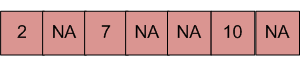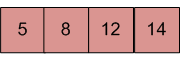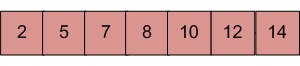# Merge an array of size n into another array of size m+n

• Difficulty Level : Easy
• Last Updated : 09 Jun, 2022

There are two sorted arrays. First one is of size m+n containing only m elements. Another one is of size n and contains n elements. Merge these two arrays into the first array of size m+n such that the output is sorted.
Input: array with m+n elements (mPlusN[]).NA => Value is not filled/available in array mPlusN[]. There should be n such array blocks.
Input: array with n elements (N[]).Output: N[] merged into mPlusN[] (Modified mPlusN[])Algorithm:

```Let first array be mPlusN[] and other array be N[]
1) Move m elements of mPlusN[] to end.
2) Start from nth element of mPlusN[] and 0th
element of N[] and merge them into mPlusN[].```

Below is the implementation of the above algorithm :

## C++

 `// C++ program to Merge an array of``// size n into another array of size m + n``#include ``using` `namespace` `std;` `/* Assuming -1 is filled for the places``   ``where element is not available */``#define NA -1` `/* Function to move m elements at``   ``the end of array mPlusN[] */``void` `moveToEnd(``int` `mPlusN[], ``int` `size)``{``   ``int` `j = size - 1;``   ``for` `(``int` `i = size - 1; i >= 0; i--)``     ``if` `(mPlusN[i] != NA)``     ``{``        ``mPlusN[j] = mPlusN[i];``        ``j--;``     ``}``}` `/* Merges array N[] of size n into``   ``array mPlusN[] of size m+n*/``int` `merge(``int` `mPlusN[], ``int` `N[], ``int` `m, ``int` `n)``{``   ``int` `i = n; ``/* Current index of i/p part of mPlusN[]*/``   ``int` `j = 0; ``/* Current index of N[]*/``   ``int` `k = 0; ``/* Current index of output mPlusN[]*/``   ``while` `(k < (m + n))``   ``{``    ``/* Take an element from mPlusN[] if``    ``a) value of the picked element is smaller``       ``and we have not reached end of it``    ``b) We have reached end of N[] */``    ``if` `((j == n)||(i < (m + n) && mPlusN[i] <= N[j]))``    ``{``        ``mPlusN[k] = mPlusN[i];``        ``k++;``        ``i++;``    ``}``    ``else` `// Otherwise take element from N[]``    ``{``       ``mPlusN[k] = N[j];``       ``k++;``       ``j++;``    ``}``   ``}``}` `/* Utility that prints out an array on a line */``void` `printArray(``int` `arr[], ``int` `size)``{``   ``for` `(``int` `i = 0; i < size; i++)``   ``cout << arr[i] << ``" "``;` `   ``cout << endl;``}` `/* Driver code */``int` `main()``{``   ``/* Initialize arrays */``   ``int` `mPlusN[] = {2, 8, NA, NA, NA, 13, NA, 15, 20};``   ``int` `N[] = {5, 7, 9, 25};``   ` `   ``int` `n = ``sizeof``(N) / ``sizeof``(N);``   ``int` `m = ``sizeof``(mPlusN) / ``sizeof``(mPlusN) - n;` `   ``/*Move the m elements at the end of mPlusN*/``   ``moveToEnd(mPlusN, m + n);` `   ``/*Merge N[] into mPlusN[] */``   ``merge(mPlusN, N, m, n);` `   ``/* Print the resultant mPlusN */``   ``printArray(mPlusN, m+n);` `   ``return` `0;``}`

## C

 `// C program to Merge an array of``// size n into another array of size m + n``#include ` `/* Assuming -1 is filled for``   ``the places where element``   ``is not available */``#define NA -1` `/* Function to move m elements``   ``at the end of array mPlusN[]`` ``*/``void` `moveToEnd(``int` `mPlusN[], ``int` `size)``{``    ``int` `i = 0, j = size - 1;``    ``for` `(i = size - 1; i >= 0; i--)``        ``if` `(mPlusN[i] != NA) {``            ``mPlusN[j] = mPlusN[i];``            ``j--;``        ``}``}` `/* Merges array N[] of size n into array mPlusN[]``   ``of size m+n*/``int` `merge(``int` `mPlusN[], ``int` `N[], ``int` `m, ``int` `n)``{``    ``int` `i = n; ``/* Current index of i/p part of mPlusN[]*/``    ``int` `j = 0; ``/* Current index of N[]*/``    ``int` `k = 0; ``/* Current index of output mPlusN[]*/``    ``while` `(k < (m + n))``    ``{``        ``/* Take an element from mPlusN[] if``           ``a) value of the picked element is smaller and we``           ``have not reached end of it``           ``b) We have reached end of N[] */``        ``if` `((j == n)|| (i < (m + n)``             ``&& mPlusN[i] <= N[j]))``        ``{``            ``mPlusN[k] = mPlusN[i];``            ``k++;``            ``i++;``        ``}``        ``else` `// Otherwise take element from N[]``        ``{``            ``mPlusN[k] = N[j];``            ``k++;``            ``j++;``        ``}``    ``}``}` `/* Utility that prints out an array on a line */``void` `printArray(``int` `arr[], ``int` `size)``{``    ``int` `i;``    ``for` `(i = 0; i < size; i++)``        ``printf``(``"%d "``, arr[i]);` `    ``printf``(``"\n"``);``}` `/* Driver code */``int` `main()``{``    ``/* Initialize arrays */``    ``int` `mPlusN[] = { 2, 8, NA, NA, NA, 13, NA, 15, 20 };``    ``int` `N[] = { 5, 7, 9, 25 };``    ``int` `n = ``sizeof``(N) / ``sizeof``(N);``    ``int` `m = ``sizeof``(mPlusN) / ``sizeof``(mPlusN) - n;` `    ``/*Move the m elements at the end of mPlusN*/``    ``moveToEnd(mPlusN, m + n);` `    ``/*Merge N[] into mPlusN[] */``    ``merge(mPlusN, N, m, n);` `    ``/* Print the resultant mPlusN */``    ``printArray(mPlusN, m + n);` `    ``return` `0;``}`

## Java

 `// Java program to Merge an array of``// size n into another array of size m + n` `class` `MergeArrays {``    ``/* Function to move m``       ``elements at the end of array``     ``* mPlusN[] */``    ``void` `moveToEnd(``int` `mPlusN[], ``int` `size)``    ``{``        ``int` `i, j = size - ``1``;``        ``for` `(i = size - ``1``; i >= ``0``; i--) {``            ``if` `(mPlusN[i] != -``1``) {``                ``mPlusN[j] = mPlusN[i];``                ``j--;``            ``}``        ``}``    ``}` `    ``/* Merges array N[] of``       ``size n into array mPlusN[]``       ``of size m+n*/``    ``void` `merge(``int` `mPlusN[], ``int` `N[], ``int` `m, ``int` `n)``    ``{``        ``int` `i = n;` `        ``/* Current index of i/p part of mPlusN[]*/``        ``int` `j = ``0``;` `        ``/* Current index of N[]*/``        ``int` `k = ``0``;` `        ``/* Current index of output mPlusN[]*/``        ``while` `(k < (m + n))``        ``{``            ``/* Take an element from mPlusN[] if``            ``a) value of the picked element is smaller and we``            ``have not reached end of it b) We have reached``            ``end of N[] */``            ``if` `((i < (m + n) && mPlusN[i] <= N[j])``                ``|| (j == n)) {``                ``mPlusN[k] = mPlusN[i];``                ``k++;``                ``i++;``            ``}``            ``else` `// Otherwise take element from N[]``            ``{``                ``mPlusN[k] = N[j];``                ``k++;``                ``j++;``            ``}``        ``}``    ``}` `    ``/* Utility that prints out an array on a line */``    ``void` `printArray(``int` `arr[], ``int` `size)``    ``{``        ``int` `i;``        ``for` `(i = ``0``; i < size; i++)``            ``System.out.print(arr[i] + ``" "``);` `        ``System.out.println(``""``);``    ``}` `    ``// Driver Code``    ``public` `static` `void` `main(String[] args)``    ``{``        ``MergeArrays mergearray = ``new` `MergeArrays();` `        ``/* Initialize arrays */``        ``int` `mPlusN[] = { ``2``, ``8``, -``1``, -``1``, -``1``, ``13``, -``1``, ``15``, ``20` `};``        ``int` `N[] = { ``5``, ``7``, ``9``, ``25` `};``        ``int` `n = N.length;``        ``int` `m = mPlusN.length - n;` `        ``/*Move the m elements at the end of mPlusN*/``        ``mergearray.moveToEnd(mPlusN, m + n);` `        ``/*Merge N[] into mPlusN[] */``        ``mergearray.merge(mPlusN, N, m, n);` `        ``/* Print the resultant mPlusN */``        ``mergearray.printArray(mPlusN, m + n);``    ``}``}` `// This code has been contributed by Mayank Jaiswal`

## Python3

 `# Python program to Merge an array of``# size n into another array of size m + n` `NA ``=` `-``1` `# Function to move m elements``# at the end of array mPlusN[]`  `def` `moveToEnd(mPlusN, size):` `    ``i ``=` `0``    ``j ``=` `size ``-` `1``    ``for` `i ``in` `range``(size``-``1``, ``-``1``, ``-``1``):``        ``if` `(mPlusN[i] !``=` `NA):` `            ``mPlusN[j] ``=` `mPlusN[i]``            ``j ``-``=` `1` `# Merges array N[]``# of size n into array mPlusN[]``# of size m+n`  `def` `merge(mPlusN, N, m, n):` `    ``i ``=` `n  ``# Current index of i/p part of mPlusN[]``    ``j ``=` `0`  `# Current index of N[]``    ``k ``=` `0`  `# Current index of output mPlusN[]``    ``while` `(k < (m``+``n)):` `        ``# Take an element from mPlusN[] if``        ``# a) value of the picked``        ``# element is smaller and we have``        ``# not reached end of it``        ``# b) We have reached end of N[] */``        ``if` `((j ``=``=` `n) ``or` `(i < (m``+``n) ``and` `mPlusN[i] <``=` `N[j])):` `            ``mPlusN[k] ``=` `mPlusN[i]``            ``k ``+``=` `1``            ``i ``+``=` `1` `        ``else``:  ``# Otherwise take element from N[]` `            ``mPlusN[k] ``=` `N[j]``            ``k ``+``=` `1``            ``j ``+``=` `1` `# Utility that prints``# out an array on a line`  `def` `printArray(arr, size):` `    ``for` `i ``in` `range``(size):``        ``print``(arr[i], ``" "``, end``=``"")` `    ``print``()`  `# Driver function to``# test above functions` `# Initialize arrays``mPlusN ``=` `[``2``, ``8``, NA, NA, NA, ``13``, NA, ``15``, ``20``]``N ``=` `[``5``, ``7``, ``9``, ``25``]``n ``=` `len``(N)` `m ``=` `len``(mPlusN) ``-` `n` `# Move the m elements``# at the end of mPlusN``moveToEnd(mPlusN, m``+``n)` `# Merge N[] into mPlusN[]``merge(mPlusN, N, m, n)` `# Print the resultant mPlusN``printArray(mPlusN, m``+``n)` `# This code is contributed``# by Anant Agarwal.`

## C#

 `// C# program to Merge an array of``// size n into another array of size m + n``using` `System;` `class` `GFG {``    ``/* Function to move m elements at``       ``the end of array mPlusN[] */``    ``public` `virtual` `void` `moveToEnd(``int``[] mPlusN, ``int` `size)``    ``{``        ``int` `i, j = size - 1;``        ``for` `(i = size - 1; i >= 0; i--)``        ``{``            ``if` `(mPlusN[i] != -1) {``                ``mPlusN[j] = mPlusN[i];``                ``j--;``            ``}``        ``}``    ``}` `    ``/* Merges array N[] of size n``       ``into array mPlusN[] of size m+n*/``    ``public` `virtual` `void` `merge(``int``[] mPlusN,``                              ``int``[] N, ``int` `m,``                              ``int` `n)``    ``{``        ``int` `i = n;` `        ``/* Current index of i/p``           ``part of mPlusN[]*/``        ``int` `j = 0;` `        ``/* Current index of N[]*/``        ``int` `k = 0;` `        ``/* Current index of output mPlusN[]*/``        ``while` `(k < (m + n))``        ``{``            ``/* Take an element from mPlusN[] if``            ``a) value of the picked element is smaller``               ``and we have not reached end of it``            ``b) We have reached end of N[] */``            ``if` `((j == n)(i < (m + n)``                ``&& mPlusN[i] <= N[j]))``            ``{``                ``mPlusN[k] = mPlusN[i];``                ``k++;``                ``i++;``            ``}``            ``else` `// Otherwise take element from N[]``            ``{``                ``mPlusN[k] = N[j];``                ``k++;``                ``j++;``            ``}``        ``}``    ``}` `    ``/* Utility that prints out``       ``an array on a line */``    ``public` `virtual` `void` `printArray(``int``[] arr, ``int` `size)``    ``{``        ``int` `i;``        ``for` `(i = 0; i < size; i++) {``            ``Console.Write(arr[i] + ``" "``);``        ``}` `        ``Console.WriteLine(``""``);``    ``}` `    ``// Driver Code``    ``public` `static` `void` `Main(``string``[] args)``    ``{``        ``GFG mergearray = ``new` `GFG();` `        ``/* Initialize arrays */``        ``int``[] mPlusN = ``new` `int``[] { 2,  8,  -1, -1, -1,``                                   ``13, -1, 15, 20 };``        ``int``[] N = ``new` `int``[] { 5, 7, 9, 25 };``        ``int` `n = N.Length;``        ``int` `m = mPlusN.Length - n;` `        ``/*Move the m elements at the``          ``end of mPlusN*/``        ``mergearray.moveToEnd(mPlusN, m + n);` `        ``/*Merge N[] into mPlusN[] */``        ``mergearray.merge(mPlusN, N, m, n);` `        ``/* Print the resultant mPlusN */``        ``mergearray.printArray(mPlusN, m + n);``    ``}``}` `// This code is contributed by Shrikant13`

## PHP

 `= 0; ``\$i``--)``        ``if` `(``\$mPlusN``[``\$i``] != ``\$NA``)``        ``{``            ``\$mPlusN``[``\$j``] = ``\$mPlusN``[``\$i``];``            ``\$j``--;``        ``}``}` `/* Merges array N[] of size n``into array mPlusN[] of size m+n*/``function` `merge(&``\$mPlusN``, &``\$N``, ``\$m``, ``\$n``)``{``    ``\$i` `= ``\$n``; ``/* Current index of i/p``                ``part of mPlusN[]*/``    ``\$j` `= 0;  ``/* Current index of N[]*/``    ``\$k` `= 0;  ``/* Current index of``                ``output mPlusN[]*/``    ``while` `(``\$k` `< (``\$m` `+ ``\$n``))``    ``{``        ``/* Take an element from mPlusN[] if``        ``a) value of the picked element``           ``is smaller and we have not``           ``reached end of it``        ``b) We have reached end of N[] */``        ``if` `((``\$j` `== ``\$n``) || (``\$i` `< (``\$m` `+ ``\$n``) &&``             ``\$mPlusN``[``\$i``] <= ``\$N``[``\$j``]))``        ``{``            ``\$mPlusN``[``\$k``] = ``\$mPlusN``[``\$i``];``            ``\$k``++;``            ``\$i``++;``        ``}``        ``else` `// Otherwise take element from N[]``        ``{``            ``\$mPlusN``[``\$k``] = ``\$N``[``\$j``];``            ``\$k``++;``            ``\$j``++;``        ``}``}``}` `/* Utility that prints out``   ``an array on a line */``function` `printArray(&``\$arr``, ``\$size``)``{``    ``for` `(``\$i` `= 0; ``\$i` `< ``\$size``; ``\$i``++)``    ``echo` `\$arr``[``\$i``] . ``" "``;``    ` `    ``echo` `"\n"``;``}` `// Driver Code` `/* Initialize arrays */``\$mPlusN` `= ``array``(2, 8, ``\$NA``, ``\$NA``, ``\$NA``,``                ``13, ``\$NA``, 15, 20);``\$N` `= ``array``(5, 7, 9, 25);``    ` `\$n` `= sizeof(``\$N``);``\$m` `= sizeof(``\$mPlusN``) - ``\$n``;` `/* Move the m elements``   ``at the end of mPlusN*/``moveToEnd(``\$mPlusN``, ``\$m` `+ ``\$n``);` `/* Merge N[] into mPlusN[] */``merge(``\$mPlusN``, ``\$N``, ``\$m``, ``\$n``);` `/* Print the resultant mPlusN */``printArray(``\$mPlusN``, ``\$m``+``\$n``);` `// This code is contributed``// by ChitraNayal``?>`

## Javascript

 ``

Output

`2 5 7 8 9 13 15 20 25 `

Time Complexity: O(m+n)

Auxiliary Space: O(1)

Please write comment if you find any bug in the above program or a better way to solve the same problem.

My Personal Notes arrow_drop_up